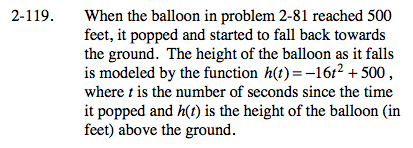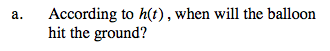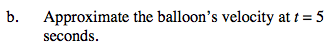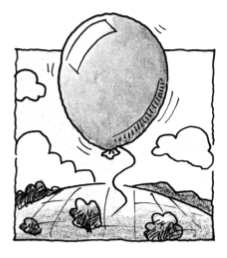### Home > CALC > Chapter Ch2 > Lesson 2.3.2 > Problem2-119

2-119.What value of h represents the ground?Velocity is slope of a distance/position graph. Since h(t) is a parabola, we do not have the skills (yet) to find the slope on a curve. But we can approximate.

Choose two times very close to t = 5. Use the function, h(t), to find the balloon's height at those times. Find the slope of the line that goes through those points. This will be a good approximation of the velocity of the balloon at t = 5.